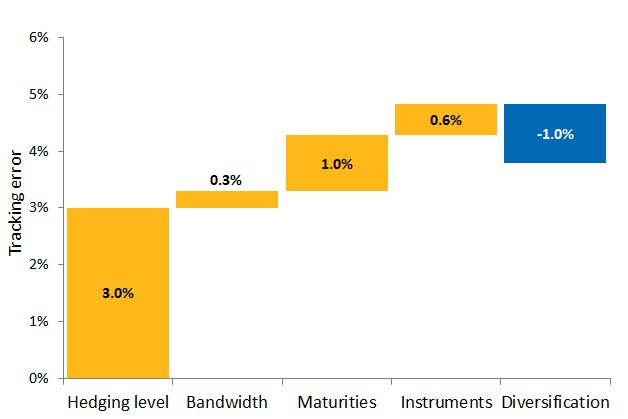# LDI Deep Dive Series (Part 5): Pulling the Pieces Together

Long-Term Liabilities

Investors with long-term liabilities, like pension funds or life insurers, are normally exposed to interest rate risk because a fall in interest rates will increase the value of their liabilities. By adopting a liability-driven investment (LDI) strategy, much of this interest rate risk can be mitigated. In this LDI Deep Dive Series, we have decomposed interest rate risk into parallel shifts of the interest rate curve, curve risk, and basis risk. This final article pulls all the pieces together and analyzes how to effectively allocate risk budget to different types of interest rate risk.

In this article, we look at the components of interest rate risk: parallel shifts of the interest rate curve, curve risk, and basis risk. Each of these risk factors contributes to funding level risk (i.e., tracking error compared to the liabilities), but might also impact the expected return. We show that carefully trading-off risk and return, while taking diversification effects into account are the ingredients for an effective LDI strategy. Due to the interaction with the liability structure, investments beliefs and other investor specific elements, an LDI strategy should, in most circumstances, be tailor-made.

We propose an iterative process for designing an LDI strategy: repeatedly checking risk-return addition of different risk factors to the most recent (draft) total portfolio until the portfolio cannot be further improved. This process results in an LDI strategy that essentially distributes the available risk budget over the risk factors that we have described in this series. This can be summarized as we do for a hypothetical pension fund in Figure 1 below. Translating the LDI strategy to a risk budget helps making clear what the most important sources of risk are and from where expected return should be expected.

##### Figure 1: Example risk budget of an LDI strategySource: Aegon Asset Management. For illustrative purposes only.